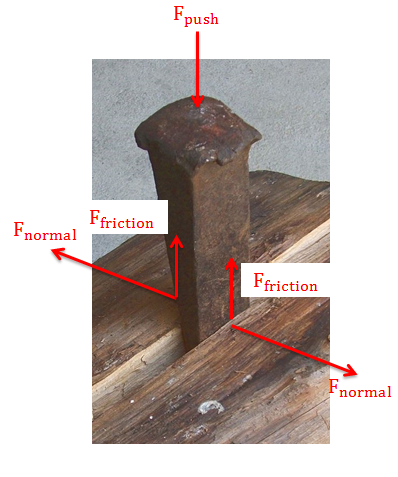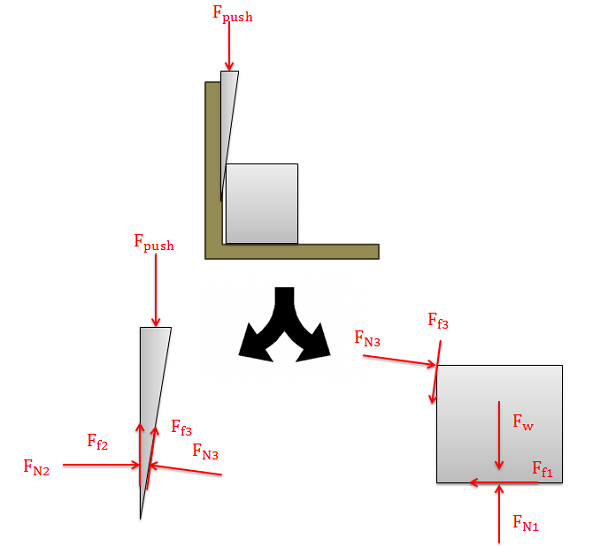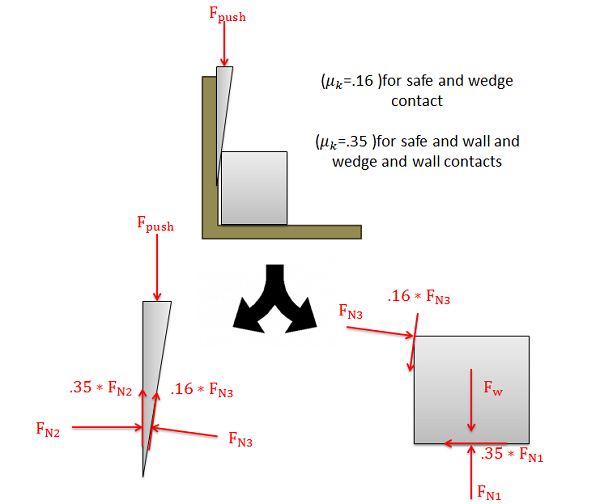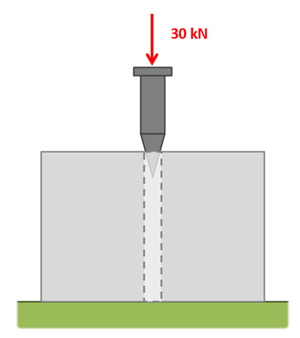﻿ Mechanics Map - Wedges

# Wedges

A wedge is a thin wedge-shaped object that is used to force two objects apart or to force one object away from a close surface. Wedges have the effect of allowing users to create very large normal forces to move objects with relatively small input forces. The friction forces in wedge systems also tend to be very large though, and can reduce the effectiveness of wedges.A hammer is used to push this wedge into the crack in this log. The normal forces are pushing the two halves of the log apart while the friction forces are opposing the pushing force. Adapted from image by Luigi Chiesa CC-BY-SA-3.0.

To analyze a wedge system we will need to draw free body diagrams of each of the bodies in the system (the wedge itself and any bodies the wedge will be moving). We need to be sure that we include the pushing force on the wedge, normal forces along any surfaces in contact, and friction forces along any surfaces in contact.The top diagram shows a wedge being used to push a safe away from a wall. The first step in analyzing the system is to draw free body diagrams of the wedge and the safe. Remember that all normal forces will be perpendicular to the surfaces in contact and that all friction forces will be parallel to the surfaces in contact.

After we draw the free body diagram we can work to simplify the problem. It is usually assumed that the wedge and the bodies will be sliding against one another, so each friction force will be equal to the kinetic coefficient of friction between the two surfaces times the associated normal force between the two forces. This reduces the number of unknowns and will usually allow us to solve for any unknown problems.By replacing each of the friction forces with the kinetic coefficient of friction times the normal force, we can reduce the number of unknowns in our analysis.

With our simplified diagram we will assume that the bodies are all in equilibrium and we will write our equilibrium equations for the two bodies. By solving the equilibrium equations, we can solve for any unknowns we have.

## Worked Problems:

### Question 1:

A heavy safe is being pushed away from a wall with a wedge as shown below. Assume the wedge has an angle of 5 degrees, the coefficient of friction (static and kinetic) between the wedge and the safe is .16, and the coefficients of friction (static and kinetic) between the wedge and the wall and the safe and the floor are both .35. What is the pushing force required to move the safe out from the wall?### Question 2:

A wedge as shown below is being used to lift the corner of the foundation of a house. How large must the pushing force be to exert a one ton (2000 lb) lifting force?